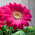### Stack implementation using Linked List

Stack is a data structure it serves as a collection of elements. It has 2 principle operation push and pop.Stack works on the principle of  Last In First Out or LIFO i.e the element that is entered last is removed first. To do this is has 2 methods
1. push() :  push operation pushes the element on the top.
2. pop() :  pop operation removes the element on the top.
This can be seen in  the below image.

Linked list is a simple linear data structure formed by collection of data elements called nodes. Each node consists of a data element and link field.The data field consists of element and link field consists of address of next element.

To know more about Stack Data Structure click here
To view Simple Linked List implementation click here

Now inorder to implement Stack using linked list we need to implement push and pop operation in Linked List. Push and pop can be implemented by using other already implemented functions of Linked List like addToStart, addToEnd and remove functions.

### Sample input and output to check the program

You might Also be interested in

Binary Search Tree
Height of Binary Search Tree

1.### Infix to Prefix conversion using Stack

This post is about conversion of Infix expression to Prefix conversion. For this conversion we take help of stack data structure, we need to push and pop the operators in and out of the stack.

Infix expressions are the expressions that we normally use,eg. 5+6-7; a+b*c etc. Prefix expressions are the expressions in which the 2 operands are preceded by the operator eg. -+567 , +a*bc etc.

This method is very similar to the method that we used to convert Infix to Postfix but the only difference is that here we need to reverse the input string before conversion and then reverse the final output string before displaying it.

NOTE: This changes one thing that is instead of encountering the opening bracket we now first encounter the closing bracket and we make changes accordingly in our code.

So, to convert an infix expression to a prefix expression we follow the below steps
(we have 2 string, 1st is the input infix expression string 2nd is the output string which is empty initially)

We first revers…

Hashing is a technique used for storing , searching and removing elements in almost constant time. Hashing is done with help of a hash function that generates index for a given input, then this index can be used to search the elements, store an element, or remove that element from that index.

A hash function is a function that is used to map the data elements to their position in the data structure used. For example if we use an array to store the integer elements then the hash function will generate position for each element so that searching, storing and removing operation on the array can be done in constant time that is independent of the number of elements in the array. For better look at the example below.

now we face a problem if for 2 numbers same position is generated example consider elements 1 and 14

1 % 13 = 1

14 % 13 = 1

so when we get 1 we store it at the first position, but when we get 14 we see that the position 1 is already taken, this is a case of collision.

Inorder…